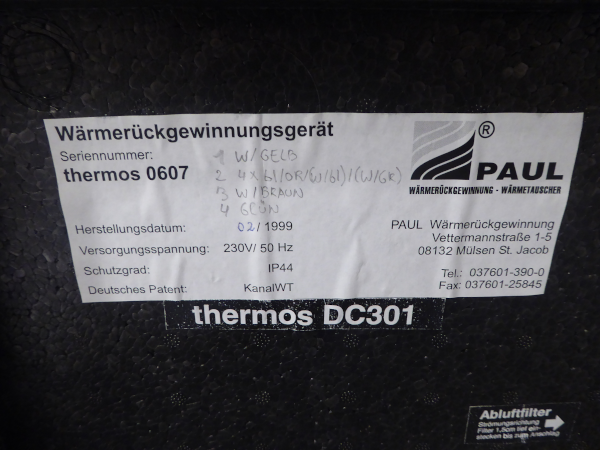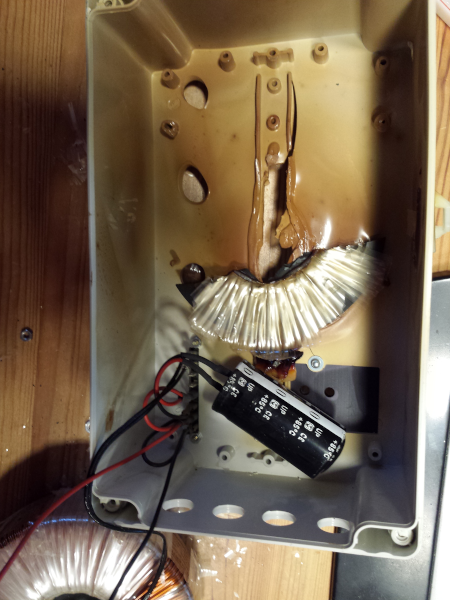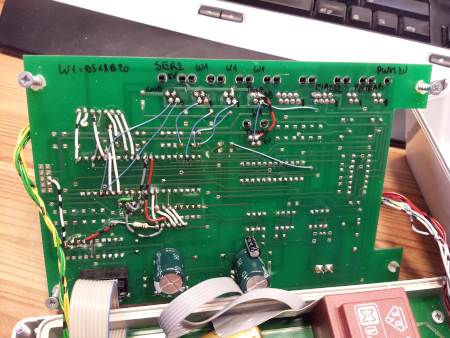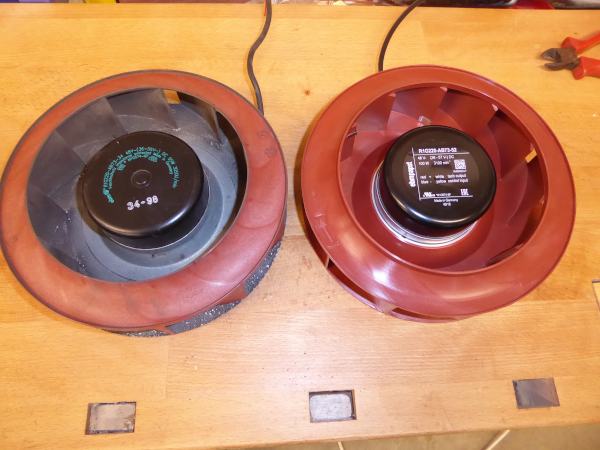# Single board computer projects

## Piventi: Refurbishing an MVHR with Raspi and Teensy using MQTT

last updated: 03/06/20

In 1999 I bought my Mechanical Ventilation Heat Recovery (MVHR) system from Paul.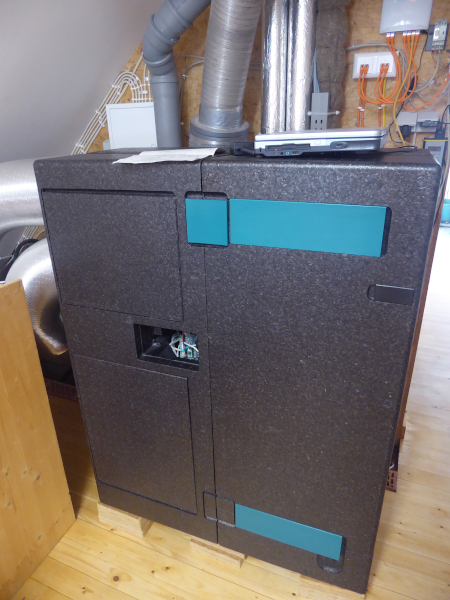I didn't like the control system so it was replaced with an AVR Atmega. In 2014 I saw, that the transformer overheated and replaced it with an 48V switching power supply. 2016 after 17 years of working one of the vents began to be noisy (pictures at the end of the page).

I decided it was time to replace the vents and wanted a system that could be managed over Ethernet.

A Raspberry Pi (Raspi) should do the MQTT publishing. The real time hardware part should be done with an Arduino (Teensy 2.0). Both are connected through I²C.

The cool thing is that the Arduino IDE runs on the Raspi and so reprogramming is really easy with the help of VNC. I added also four temperature and humidity sensors, a temperature sensor for the housing and an CO2 sensor.

The MVHR is now sending MQTT messages in JSON format: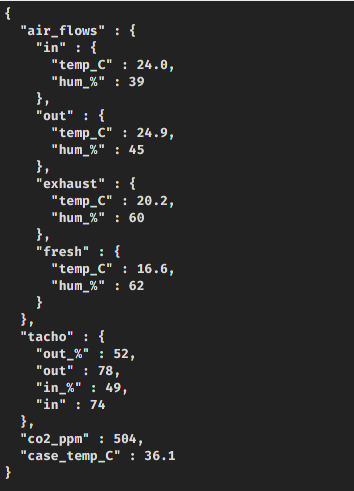and with the following JSON string we can change the flow-rate: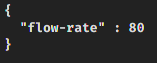This can be done e.g. with the `Pitoucon` device.

### Hardware

An MHVR is quite a simple system if PWM controlled ventilators are used. I'm refurbishing here my old MVHR. But another solution used for a school project was to buy a system without electronics and to built the electronics needed.

#### BOM

• Raspberry Pi to do the MQTT communication and to reprogram the Teensy
• A Teensy 2.0 connected through USB with the Raspi is programmed with the Arduino IDE (Teensyduino) running on the Raspi.
• The ventilators, model `R1G220-AB73`, are from ebmpapst and use a PWM to be controlled. A feedback of the regulated motors is delivered b y a tacho signal.
• 4 Sensirion SHT75 connected to the Teensy are used to measure temperatures and the humidity of the the 4 airflows (in, out, exhaust and fresh air).
• A K30 CO2 sensor from senseair, connected through a serial interface with the Raspi, measures the CO2 value of the exhaust air.
• A DS18B20 temperature sensor connected to the Raspi measures the temperature in the housing.

#### Circuit### Software

I choose the Raspi OS image with desktop, so it is possible to enable VNC and administrate the piventi (and reprogram the Raspi or the Teensy) from every computer in the network. For MQTT we need to install the Python library:

``````    sudo apt install python3-pip
sudo python3 -m pip install paho-mqtt
``````

Run these commands in terminal to update your raspi and to make a folder named `arduino` in your home directory:

``````    sudo apt update
mkdir ~/arduino
``````

``````    cd ~/arduino/arduino-1.8.12
sudo ./install.sh
``````

Download the newest Teensyduino version (ARM version!!) on pjrc.com. Make the file executable and run it. Choose the right directory with your arduino (e. g. ~/arduino/arduino-1.8.12).

To set up the udev rules (so you have not to be root to use your Teensy), use this file: udev rules and copy it to `/etc/udev/rules.d`. Restart your raspi ()`sudo reboot`).

#### Start at boot

To start the script, add the following line to your `/etc/rc.local` file (sleep waits for the network) before the exit line:

``````    (sleep 10 #wait for network
python3 /home/pi/piventi/piventi.py) &
``````

I use ethernet but if you want to use WiFi add also the following line to `/etc/rc.local`:

``````    /sbin/iw dev wlan0 set power_save off
``````

#### Teensy software

The Teensy software (download at the end of the page) creates an I2C slave and gets the data from the 4 temperature and humidity sensors. It also sends the PWM signal to the two vents and gets the two Tacho signals using pin change interrupts.

``````    // piventi.ino weigu.lu

#include <Wire.h>
#include "Sensirion.h"

#ifndef cbi
#define cbi(sfr, bit) (_SFR_BYTE(sfr) &= ~_BV(bit))
#endif

Sensirion sht1 = Sensirion(2, 1);   // clk1  PB1, data1 PB2
Sensirion sht2 = Sensirion(3, 4);   // clk2  PB7, data2 PB3
Sensirion sht3 = Sensirion(9, 12);   // clk3  PD7, data3 PC6
Sensirion sht4 = Sensirion(16, 13); // clk4  PB4, data4 PF7

// exhaust
const byte fan1Pin = 10;    // PC7 on Teensy (OC4A of Timer 4)
const byte Tacho1Pin = 14;  // PB5 on Teensy (PCINT5)
const byte PCINTPinT1 = 5;  // PCINT5
// fresh
const byte fan2Pin = 15;    // PB6 on Teensy (OC4B of Timer 4)
const byte Tacho2Pin = 0;   // PB0 on Teensy (PCINT0)
const byte PCINTPinT2 = 0;  // PCINT0

const byte teensyLED = 11; // PD6 Teensy LED

volatile byte oldPort = 0x00;
volatile byte T1Cnt = 0, T2Cnt = 0;
volatile byte T1CntVal = 0, T2CntVal = 0;
byte volatile Command, Control, Sensor, Tacho;
byte volatile txTable, rxTable;

struct {
float t1, t2, t3, t4;
float h1,h2,h3,h4;
float dp1,dp2,dp3,dp4;
float h371,h372,h373,h374;
int ret1,ret2,ret3,ret4;
} t;

struct {
float volatile t1, t2, t3, t4;
float volatile h1,h2,h3,h4;
float volatile dp1,dp2,dp3,dp4;
float volatile h371,h372,h373,h374;
} data;

void setup() {
Command = 0;
Control = 0;
Sensor = 0;
Tacho = 0;
OCR4A = 127; // both vents on 50%
OCR4B = 127; // inverted because of Transistor!
pinMode(teensyLED, OUTPUT);
pinMode(fan1Pin, OUTPUT);
pinMode(fan2Pin, OUTPUT);
// Fast PWM for Teensy Timer 4
TCCR4A = _BV(COM4A1) | _BV(COM4B1) | _BV(PWM4A) | _BV(PWM4B);
TCCR4B = _BV(CS42); //3,9kHz
cbi(PORTD, 0); // no internal PU
cbi(PORTD, 1); // Raspi has 2x1,8k
Wire.onRequest(i2cTransmit); // i2c interrupt send
pinMode(Tacho1Pin, INPUT_PULLUP);
pinMode(Tacho2Pin, INPUT_PULLUP);
attachPinChangeInterrupt();
}

void loop() {
while (!(t.ret1 == S_Meas_Rdy)) {// A new measurement is available
t.ret1 = sht1.measure(&t.t1, &t.h1, &t.dp1, 37, &t.h371);
}
data.t1 = t.t1;
data.h1 = t.h1;
data.dp1 = t.dp1;
data.h371 = t.h371;
t.ret1 = 0;
while (!(t.ret2 == S_Meas_Rdy)) {
t.ret2 = sht2.measure(&t.t2, &t.h2, &t.dp2, 37, &t.h372);} // Measurement
data.t2 = t.t2;
data.h2 = t.h2;
data.dp2 = t.dp2;
data.h372 = t.h372;
t.ret2 = 0;
while (!(t.ret3 == S_Meas_Rdy)) {
t.ret3 = sht3.measure(&t.t3, &t.h3, &t.dp3, 37, &t.h373);} // Measurement
data.t3 = t.t3;
data.h3 = t.h3;
data.dp3 = t.dp3;
data.h373 = t.h373;
t.ret3 = 0;
while (!(t.ret4 == S_Meas_Rdy)) {
t.ret4 = sht4.measure(&t.t4, &t.h4, &t.dp4, 37, &t.h374);} // Measurement
data.t4 = t.t4;
data.h4 = t.h4;
data.dp4 = t.dp4;
data.h374 = t.h374;
t.ret4 = 0;
digitalWrite(teensyLED,LOW);
T1Cnt = 0;
T2Cnt = 0;
for (unsigned int i=0; i <= 100; i++) delayMicroseconds(10000); // measure during 1s
T1CntVal = T1Cnt;
T2CntVal = T2Cnt;
digitalWrite(teensyLED,HIGH);
for (unsigned int i=0; i <= 10; i++) delayMicroseconds(10000); // 100ms*/
}

while(1 < Wire.available()) {
}
switch (Command) {          // parse vent commands (0x1n, 0x2n)
case 0x10:
OCR4A = 255;  // off
break;
case 0x11:
OCR4A = 1;    // 100%
break;
case 0x12:
OCR4A = 225;  // 20%
break;
case 0x13:
OCR4A = 215;  // 30%
break;
case 0x14:
OCR4A = 200;  // 40%
break;
case 0x15:
OCR4A = 178;  // 50%
break;
case 0x16:
OCR4A = 153;  // 60%
break;
case 0x17:
OCR4A = 130;  // 70%
break;
case 0x18:
OCR4A = 90;   // 80%
break;
case 0x19:
OCR4A = 60;   // 90%
break;
case 0x20:
OCR4B = 255;  // off
break;
case 0x21:
OCR4B = 1;    // 100%
break;
case 0x22:
OCR4B = 225;  // 20%
break;
case 0x23:
OCR4B = 215;  // 30%
break;
case 0x24:
OCR4B = 200;  // 40%
break;
case 0x25:
OCR4B = 178;  // 50%
break;
case 0x26:
OCR4B = 153;  // 60%
break;
case 0x27:
OCR4B = 130;  // 70%
break;
case 0x28:
OCR4B = 90;   // 80%
break;
case 0x29:
OCR4B = 60;   // 90%
break;
case 0x31:
Sensor = 1;
Control = 8;
break;
case 0x32:
Sensor = 2;
Control = 8;
break;
case 0x33:
Sensor = 3;
Control = 8;
break;
case 0x34:
Sensor = 4;
Control = 8;
break;
case 0x41:
Tacho = 1;
Control = 1;
break;
case 0x42:
Tacho = 2;
Control = 1;
break;
default:
break;
}
Command = 0;
}

void i2cTransmit() {
int tmp1 = 0; // temporary variable used in switch occasionally below
if (Control == 0) return;
Control -= 1;
switch (Sensor) {
case 1: //
tmp1 = int(round(data.t1 * 100));
txTable = (byte)(tmp1 & 0xFF);
txTable = (byte)(tmp1 >> 8);
tmp1 = int(round(data.h1 * 100));
txTable = (byte)(tmp1 & 0xFF);
txTable = (byte)(tmp1 >> 8);
tmp1 = int(round(data.dp1 * 100));
txTable = (byte)(tmp1 & 0xFF);
txTable = (byte)(tmp1 >> 8);
tmp1 = int(round(data.h371 * 100));
txTable = (byte)(tmp1 & 0xFF);
txTable = (byte)(tmp1 >> 8);
break;
case 2: //
tmp1 = int(round(data.t2 * 100));
txTable = (byte)(tmp1 & 0xFF);
txTable = (byte)(tmp1 >> 8);
tmp1 = int(round(data.h2 * 100));
txTable = (byte)(tmp1 & 0xFF);
txTable = (byte)(tmp1 >> 8);
tmp1 = int(round(data.dp2 * 100));
txTable = (byte)(tmp1 & 0xFF);
txTable = (byte)(tmp1 >> 8);
tmp1 = int(round(data.h372 * 100));
txTable = (byte)(tmp1 & 0xFF);
txTable = (byte)(tmp1 >> 8);
break;
case 3: //
tmp1 = int(round(data.t3 * 100));
txTable = (byte)(tmp1 & 0xFF);
txTable = (byte)(tmp1 >> 8);
tmp1 = int(round(data.h3 * 100));
txTable = (byte)(tmp1 & 0xFF);
txTable = (byte)(tmp1 >> 8);
tmp1 = int(round(data.dp3 * 100));
txTable = (byte)(tmp1 & 0xFF);
txTable = (byte)(tmp1 >> 8);
tmp1 = int(round(data.h373 * 100));
txTable = (byte)(tmp1 & 0xFF);
txTable = (byte)(tmp1 >> 8);
break;
case 4: //
tmp1 = int(round(data.t4 * 100));
txTable = (byte)(tmp1 & 0xFF);
txTable = (byte)(tmp1 >> 8);
tmp1 = int(round(data.h4 * 100));
txTable = (byte)(tmp1 & 0xFF);
txTable = (byte)(tmp1 >> 8);
tmp1 = int(round(data.dp4 * 100));
txTable = (byte)(tmp1 & 0xFF);
txTable = (byte)(tmp1 >> 8);
tmp1 = int(round(data.h374 * 100));
txTable = (byte)(tmp1 & 0xFF);
txTable = (byte)(tmp1 >> 8);
break;
default:
break; }
if (Tacho == 1) { txTable = T1CntVal; }
else {
if (Tacho == 2) { txTable = T2CntVal;}}
Wire.write(txTable[Control]); }

void attachPinChangeInterrupt(void) {
oldPort = PINB;
// pin change mask registers decide which pins are enabled as triggers
PCMSK0 |= (1 << PCINTPinT1); //PCINT0
PCMSK0 |= (1 << PCINTPinT2); //PCINT5
// PCICR: Pin Change Interrupt Control Register - enables interrupt vectors
PCICR |= (1 << PCIE0); }

ISR(PCINT0_vect) {
byte newPort = PINB;             // get the new pin states
byte change = newPort ^ oldPort; // xor to detect a rising or falling
// check which pins are triggered, compared with the settings
byte rising = change & newPort;
byte mask1 = (1 << PCINTPinT1);
byte mask2 = (1 << PCINTPinT2);
oldPort = newPort; }  // save the new state for next comparison
``````

#### Raspi software

The Python script (download at the end of the page) creates an I2 master and uses the paho-mqtt library to send and get (callback) MQTT messages. It also reads the CO2 sensor and the temperature sensor measuring the housing temperature.

``````    #!/usr/bin/env python3
# -*- coding: utf-8 -*-

from smbus import SMBus
from time import sleep
import serial
import glob
import json
import paho.mqtt.client as mqtt

port = 1 # (0 for rev.1, 1 for rev 2!)
bus = SMBus(port)

ser = serial.Serial("/dev/ttyAMA0",baudrate =9600,timeout = .5)
ser.flushInput()
sleep(1)

deviceFolder = glob.glob('/sys/bus/w1/devices/28*')  # find the file
deviceFolderString = deviceFolder
deviceFile = deviceFolderString + '/w1_slave'

clientID = "tzgtzhju"
brokerIP = "192.168.1.111"
brokerPort = 1883
topic  = "myhouse/ventilation"
DEBUG=0

def onConnect(client, userdata, flags, rc):
if DEBUG: print("Connected with result code " + str(rc))
mqttc.subscribe(topic, 0)  # Subscribe to the topic (topic name, QoS)

def onDisconnect(client, userdata, message):
if DEBUG: print("Disconnected from the broker.")

def onSubscribe(client, userdata, mid, granted_qos):
if DEBUG: print('Subscribed on topic.')

def onUnsubscribe(client, userdata, mid, granted_qos):
if DEBUG: print('Unsubscribed on topic.')

def onMessage(client, userdata, message):
if (io[0:4] != "{\"in"): # ignore own message on same topic
try:
flow = int(ioj["flow-rate"])
if (flow/10) in range(2,10):
c = flow//10
if flow == 100:
c = 1
if flow > 100:
c = 70
print(c)
sleep(0.001)
sleep(0.001)
except:
print("onmessage loop not executed (json error or I2C I/O error?)")

try:
f = open(deviceFile, 'r')
except IOError:
print('IOError')
f.close()
pos = line2.find('t=')
if pos != -1:
tempString = line2[pos + 2:]
temp = round(float(tempString) / 1000.0, 1)
else:
print('error')
return temp

# MAIN

mqttc = mqtt.Client(client_id=clientID, clean_session=True) # create client

mqttc.on_connect      = onConnect   # define the callback functions
mqttc.on_disconnect   = onDisconnect
mqttc.on_subscribe    = onSubscribe
mqttc.on_unsubscribe  = onUnsubscribe
mqttc.on_message      = onMessage

mqttc.connect(brokerIP, brokerPort, keepalive=60, bind_address="") # connect to the broker
mqttc.loop_start() # start loop to process callbacks! (new thread!)

try:
sleep(0.001)
sleep(0.001)
except:
print("OSError occured: 121 RemoteI/O error")

try:
while (True):
SENS=["","","","","","","",""]
TACHO=["","","",""]
for i in range(1,5):
try:
STemp = str(round(((rd1*256+rd2)/100),1))
if i==1:
print ("inflow: ", end='')
elif i==2:
print ("outflow: ", end='')
elif i==3:
print ("exhaust: ", end='')
else:
print ("fresh: ", end='')
print ("temp: " + STemp, end='')
SHum = str(round((rd1*256+rd2)/100))
print ("°C hum: " + SHum, end='')
print ("%")
SENS[(i-1)*2]= STemp
SENS[(i-1)*2+1]= SHum
except:
continue
for i in range(1,3): # 3 pulses/rotation, speed 3100/min = 5,7*3 = 155 meas: 151
try:
Tacho = str(rd1)
Tachop = str(round(rd1*100/151))
if i==1:
print ("tacho out: ", end='')
else:
print ("tacho in:  ", end='')
print(Tachop, end='')
print ("%  ", end='')
print(Tacho)
TACHO[(i-1)*2] = Tachop
TACHO[(i-1)*2+1] = Tacho
except:
continue
try:
ser.flushInput()
ser.write(b"\xFE\x44\x00\x08\x02\x9F\x25")
sleep(.5)
co2 = (resp*256) + resp
CO2 = str(co2)
print ("CO2 in ppm: " + CO2)
sleep(0.1)
except:
continue
print("vent housing temp in °C:",ATEMP)
sleep(60)
print('\n')
mqttc.publish(topic,'{"air_flows":{"in":{"temp_C":' + SENS +
',"hum_%":' + SENS + '},"out":{"temp_C":' +
SENS + ',"hum_%":' + SENS + '},"exhaust":'
'{"temp_C":' + SENS + ',"hum_%":' + SENS +
'},"fresh":{"temp_C":' + SENS + ',"hum_%":' +
SENS + '}},"tacho":{"out_%":' + TACHO +
',"out":' + TACHO + ',"in_%":' + TACHO +
',"in":' + TACHO + '},"co2_ppm":' + CO2 +
',"case_temp_C":' + ATEMP + '}')

except KeyboardInterrupt:
print("Keyboard interrupt by user")
``````